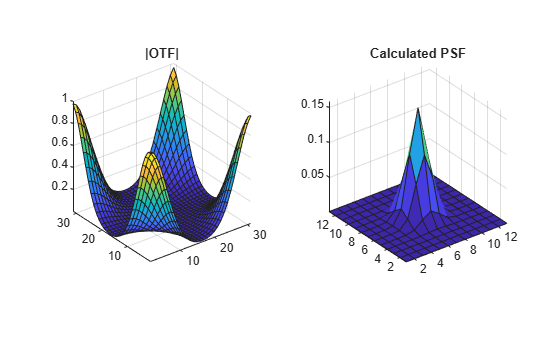# otf2psf

Convert optical transfer function to point-spread function

## Syntax

``PSF = otf2psf(OTF)``
``PSF = otf2psf(OTF,sz)``

## Description

example

````PSF = otf2psf(OTF)` computes the inverse Fast Fourier Transform of the optical transfer function (`OTF`) and creates a point-spread function (`PSF`), centered at the origin.```
````PSF = otf2psf(OTF,sz)` specifies the size, `sz`, of the output point-spread function.```

## Examples

collapse all

`PSF = fspecial('gaussian',13,1);`

Convert the PSF to an Optical Transfer Function (OTF).

`OTF = psf2otf(PSF,[31 31]);`

Convert the OTF back to a PSF.

`PSF2 = otf2psf(OTF,size(PSF));`

Plot the PSF and the OTF.

```subplot(1,2,1) surf(abs(OTF)) title('|OTF|'); axis square axis tight subplot(1,2,2) surf(PSF2) title('Corresponding PSF'); axis square axis tight```## Input Arguments

collapse all

Optical transfer function, specified as a numeric array of any dimension.

Data Types: `single` | `double` | `int8` | `int16` | `int32` | `int64` | `uint8` | `uint16` | `uint32` | `uint64`
Complex Number Support: Yes

Size of the output point-spread function `PSF`, specified as a vector of positive integers. The size of `PSF` must not exceed the size of `OTF` in any dimension. By default, `PSF` is the same size as `OTF`.

Data Types: `double`

## Output Arguments

collapse all

Point-spread function, centered at the origin, returned as a numeric array of size `sz`.

Data Types: `double`
Complex Number Support: Yes

## Tips

• To center the PSF at the origin, `otf2psf` circularly shifts the values of the output array down (or to the right) until the (1,1) element reaches the central position, then it crops the result to match dimensions specified by `sz`.

• This function is used in image convolution and deconvolution when the operations involve the FFT.

## Version History

Introduced before R2006a